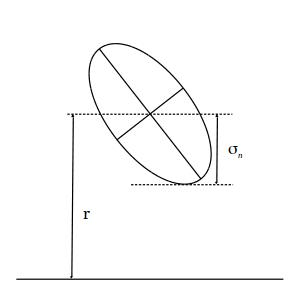$$\renewcommand{\AA}{\text{Å}}$$

# fix wall/region/ees command

## Syntax

fix ID group-ID style args

• ID, group-ID are documented in fix command

• style = wall/ees or wall/region/ees

args for style wall/ees: one or more face parameters groups may be appended
face = xlo or xhi or ylo or yhi or zlo or zhi
parameters = coord epsilon sigma cutoff
coord = position of wall = EDGE or constant or variable
EDGE = current lo or hi edge of simulation box
constant = number like 0.0 or -30.0 (distance units)
variable = equal-style variable like v_x or v_wiggle
epsilon = strength factor for wall-particle interaction (energy or energy/distance^2 units)
epsilon can be a variable (see below)
sigma = size factor for wall-particle interaction (distance units)
sigma can be a variable (see below)
cutoff = distance from wall at which wall-particle interaction is cut off (distance units)
args for style wall/region/ees: region-ID epsilon sigma cutoff
region-ID = region whose boundary will act as wall
epsilon = strength factor for wall-particle interaction (energy or energy/distance^2 units)
sigma = size factor for wall-particle interaction (distance units)
cutoff = distance from wall at which wall-particle interaction is cut off (distance units)

## Examples

fix wallhi all wall/ees xlo -1.0 1.0 1.0 2.5 units box
fix wallhi all wall/ees xhi EDGE 1.0 1.0 2.5
fix wallhi all wall/ees v_wiggle 23.2 1.0 1.0 2.5
fix zwalls all wall/ees zlo 0.0 1.0 1.0 0.858 zhi 40.0 1.0 1.0 0.858

fix ees_cube all wall/region/ees myCube 1.0 1.0 2.5


## Description

Fix wall/ees bounds the simulation domain on one or more of its faces with a flat wall that interacts with the ellipsoidal atoms in the group by generating a force on the atom in a direction perpendicular to the wall and a torque parallel with the wall. The energy of wall-particle interactions E is given by:

$E = \epsilon \left[ \frac{2 \sigma_{LJ}^{12} \left(7 r^5+14 r^3 \sigma_{n}^2+3 r \sigma_{n}^4\right) }{945 \left(r^2-\sigma_{n}^2\right)^7} -\frac{ \sigma_{LJ}^6 \left(2 r \sigma_{n}^3+\sigma_{n}^2 \left(r^2-\sigma_{n}^2\right)\log{ \left[\frac{r-\sigma_{n}}{r+\sigma_{n}}\right]}\right) }{12 \sigma_{n}^5 \left(r^2-\sigma_{n}^2\right)} \right]\qquad \sigma_n < r < r_c$

Introduced by Babadi and Ejtehadi in (Babadi). Here, r is the distance from the particle to the wall at position coord, and Rc is the cutoff distance at which the particle and wall no longer interact. Also, $$\sigma_n$$ is the distance between center of ellipsoid and the nearest point of its surface to the wall as shown below.Details of using this command and specifications are the same as fix/wall command. You can also find an example in USER/ees/ under examples/ directory.

The prefactor $$\epsilon$$ can be thought of as an effective Hamaker constant with energy units for the strength of the ellipsoid-wall interaction. More specifically, the $$\epsilon$$ prefactor is

$8 \pi^2 \quad \rho_{wall} \quad \rho_{ellipsoid} \quad \epsilon \quad \sigma_a \quad \sigma_b \quad \sigma_c$

where $$\epsilon$$ is the LJ energy parameter for the constituent LJ particles and $$\sigma_a$$, $$\sigma_b$$, and $$\sigma_c$$ are the radii of the ellipsoidal particles. $$\rho_{wall}$$ and $$\rho_{ellipsoid}$$ are the number density of the constituent particles, in the wall and ellipsoid respectively, in units of 1/volume.

Note

You must ensure that r is always bigger than $$\sigma_n$$ for all particles in the group, or LAMMPS will generate an error. This means you cannot start your simulation with particles touching the wall position coord ($$r = \sigma_n$$) or with particles penetrating the wall ($$0 =< r < \sigma_n$$) or with particles on the wrong side of the wall ($$r < 0$$).

Fix wall/region/ees treats the surface of the geometric region defined by the region-ID as a bounding wall which interacts with nearby ellipsoidal particles according to the EES potential introduced above.

Other details of this command are the same as for the fix wall/region command. One may also find an example of using this fix in the examples/PACKAGES/ees/ directory.

## Restart, fix_modify, output, run start/stop, minimize info

No information about these fixes are written to binary restart files.

The fix_modify energy option is supported by these fixes to add the energy of interaction between atoms and all the specified walls or region wall to the global potential energy of the system as part of thermodynamic output. The default settings for these fixes are fix_modify energy no.

The fix_modify respa option is supported by these fixes. This allows to set at which level of the r-RESPA integrator the fix is adding its forces. Default is the outermost level.

These fixes computes a global scalar and a global vector of forces, which can be accessed by various output commands. See the fix wall command for a description of the scalar and vector.

No parameter of these fixes can be used with the start/stop keywords of the run command.

The forces due to these fixes are imposed during an energy minimization, invoked by the minimize command.

Note

If you want the atom/wall interaction energy to be included in the total potential energy of the system (the quantity being minimized), you MUST enable the fix_modify energy option for this fix.

## Restrictions

These fixes are part of the EXTRA-FIX package. They are only enabled if LAMMPS was built with that package. See the Build package page for more info.

These fixes requires that atoms be ellipsoids as defined by the atom_style ellipsoid command.

none